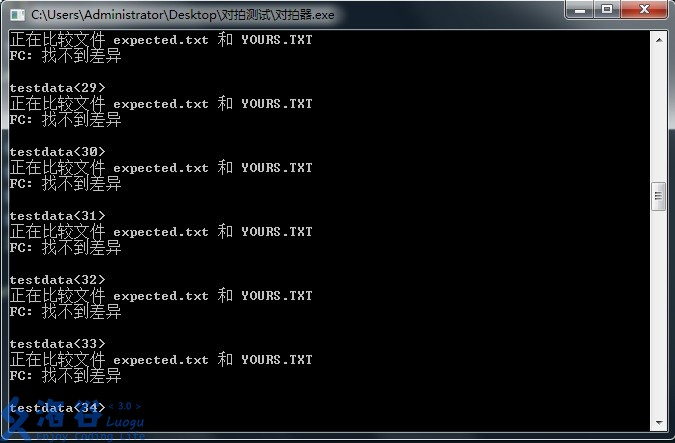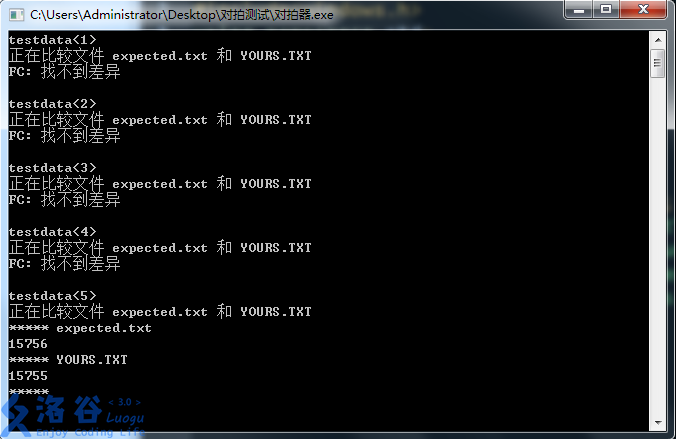# 常见技巧

## IO优化

（限于作者水平，本文仅讲述当下被称作“龟速快读”的OI优化）

## 读入优化：

int get() {
int x = 0, f = -1; char c = getchar();
while(!isdigit(c)) { if(c == '-') f = -1; c = getchar(); }
while(isdigit(c)) { x = x * 10 + c - '0'; c = getchar(); }
return x * f;
}

• 第一行：变量，c是字符，读入数字减去'0'的ASCII码值，x是读入数的绝对值，f是符号
• 第二行：吃掉多余字符，并且在其中识别出'-'号（!isdigit()表示不是数位）
• 第三行：读入数字，举个例子，现在x=123，我们接受到了一个字符c='4'，下一个x就是10x+c-'0'，即1234
• 最后返回一个带有符号的数
• 当然前面的int可以换成template<typename T>这类东西

我们在对它进行一些更改，使得它能够读入实数

double getlf(){
double x = 0, y = 1.0; int f = 1; char c = getchar();
while(!isdigit(c)) { if(c == '-') f = -1; c = getchar(); }
while(isdigit(c)) { x = x * 10 + c - '0'; c = getchar(); }
if(c != '.') return x * f;
c=getchar();
while(isdigit(c)) { x += (y /= 10) * (c - '0'); c = getchar(); }
return x * f;
}

注意第五行不能省去，因为如果给出一组这样的数据：1 2，会被读成1.2

## 输出优化：

警告：这里可能是负优化！

原理：同样，putchar比其他函数更快

代码：

void write(int x)
{
if(x<0) putchar('-'),x=-x;
if(x>9) write(x/10);
putchar(x%10+'0');
}

这个是用递归实现的。流程大概如下：

• 有一个数1234需要输出：
• 目前的范围：
• 下一层调用：4
• 下一层调用：34
• 下一层调用：234，此时的x=1，已经小于9了，所以输出'1'
• 返回上一层：输出'2'
• 以此类推，输出'3'，'4'，合起来就是1234了，输出完毕

输出实数太毒瘤了，所以这里就不写了

## 对拍

在OI中，我们常常需要将正解和暴力优势互补，暴力慢，正解的正确性有时懒得证，于是我们干脆直接将它们对比。

但是

一组一组手敲数据真的慢，还不一定查得出来

所以，我们就需要对拍

对拍四要素：

• 正解
• 暴力
• 数据生成器
• 对拍器

正解、暴力根据题目。

数据生成器：根据题目要求随机生成数据。但是可能造不出HACK数据

对拍器：将两个程序对比的机器。它的流程为：

• 生成一组数据
• 正解、暴力算结果
• 对比，判断是否一样
• 重复执行以上过程直到出错

这就要依靠<windows.h>库中的system()函数。

代码：

#include<iostream>
#include<windows.h>
using namespace std;
int main()
{
while(1)
{
system("数据生成器.exe > data.txt");
system("正解.exe < data.txt > yours.txt");
system("暴力.exe < data.txt > expected.txt");
if(system("fc expected.txt yours.txt"))   break;
}
cout<<"发现错误，请查看！"<<endl;
system("pause");
return 0;
}


例如我们要检查$$\text{BigInt A+B}$$的正确性。

数据生成器：

#include<iostream>
#include<bits/stdc++.h>
using namespace std;

int main()
{
srand(time(NULL));
int a=rand();
int b=rand();
cout<<(int)a<<" "<<(int)b;
}


两个程序：

#include<iostream>
using namespace std;

int main()
{
int a,b;
cin>>a>>b;
cout<<a+b<<endl;
}

大整数程序略

对拍器：

#include<iostream>
#include<windows.h>
using namespace std;
int main()
{
int T=0;
while(1)
{
T++;
cout<<"testdata<"<<T<<">"<<endl;
system("datamaker.exe > data.txt");
system("A+B.exe < data.txt > yours.txt");
system("A+B_1.exe < data.txt > expected.txt");
if(system("fc expected.txt yours.txt")) return 0;
}
return 0;
}


如果没有错，运行下来是这样的：我们把BigInt的输出改为：cout<<a+b+rand()%2;

考验rp的时候到了。事实证明我还是太非了，才五组就挂了。

答：“我不会。”

这部分有待补充